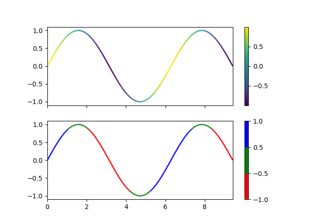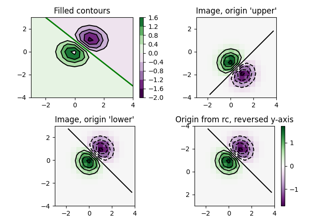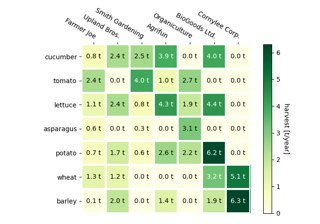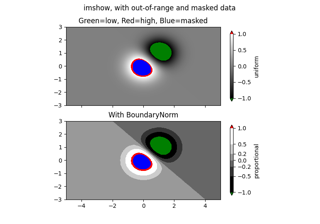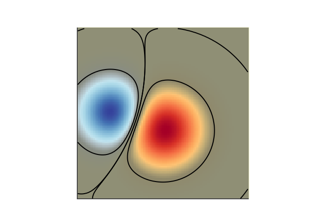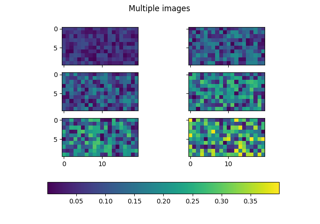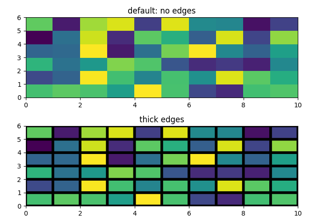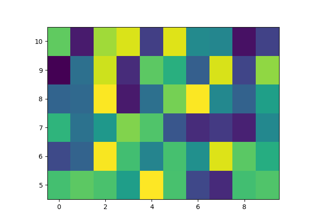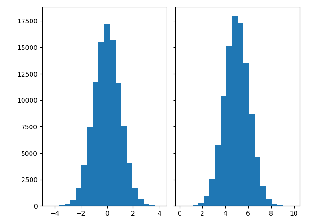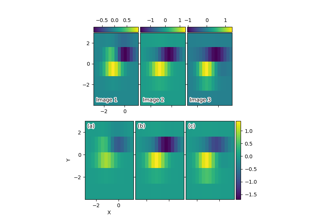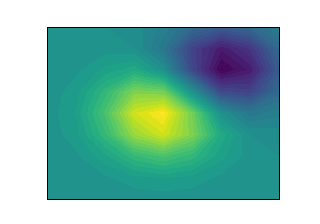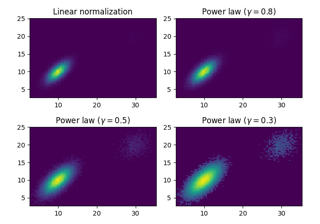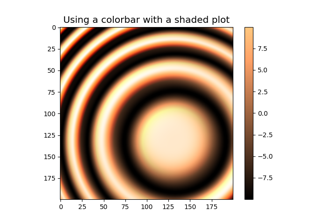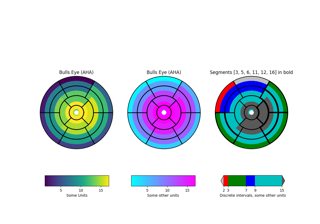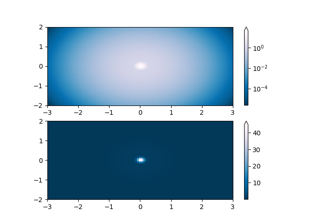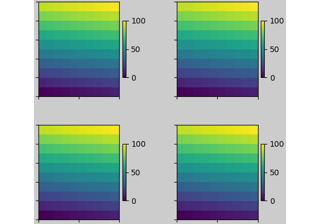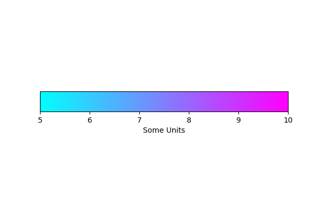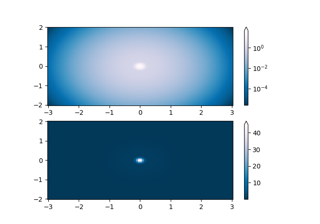matplotlib.colors.Normalize¶

class matplotlib.colors.Normalize(vmin=None, vmax=None, clip=False)[source]

Bases: object

A class which, when called, linearly normalizes data into the [0.0, 1.0] interval.

Parameters: vmin, vmaxfloat or NoneIf vmin and/or vmax is not given, they are initialized from the minimum and maximum value, respectively, of the first input processed; i.e., __call__(A) calls autoscale_None(A). clipbool, default: FalseIf True values falling outside the range [vmin, vmax], are mapped to 0 or 1, whichever is closer, and masked values are set to 1. If False masked values remain masked. Clipping silently defeats the purpose of setting the over, under, and masked colors in a colormap, so it is likely to lead to surprises; therefore the default is clip=False.

Notes

Returns 0 if vmin == vmax.

__call__(self, value, clip=None)[source]

Normalize value data in the [vmin, vmax] interval into the [0.0, 1.0] interval and return it.

Parameters: valueData to normalize. clipboolIf None, defaults to self.clip (which defaults to False).

Notes

If not already initialized, self.vmin and self.vmax are initialized using self.autoscale_None(value).

__dict__ = mappingproxy({'__module__': 'matplotlib.colors', '__doc__': '\n A class which, when called, linearly normalizes data into the\n [0.0, 1.0] interval.\n ', '__init__': <function Normalize.__init__>, 'process_value': <staticmethod object>, '__call__': <function Normalize.__call__>, 'inverse': <function Normalize.inverse>, 'autoscale': <function Normalize.autoscale>, 'autoscale_None': <function Normalize.autoscale_None>, 'scaled': <function Normalize.scaled>, '__dict__': <attribute '__dict__' of 'Normalize' objects>, '__weakref__': <attribute '__weakref__' of 'Normalize' objects>, '__slotnames__': []})
__init__(self, vmin=None, vmax=None, clip=False)[source]
Parameters: vmin, vmaxfloat or NoneIf vmin and/or vmax is not given, they are initialized from the minimum and maximum value, respectively, of the first input processed; i.e., __call__(A) calls autoscale_None(A). clipbool, default: FalseIf True values falling outside the range [vmin, vmax], are mapped to 0 or 1, whichever is closer, and masked values are set to 1. If False masked values remain masked. Clipping silently defeats the purpose of setting the over, under, and masked colors in a colormap, so it is likely to lead to surprises; therefore the default is clip=False.

Notes

Returns 0 if vmin == vmax.

__module__ = 'matplotlib.colors'
__slotnames__ = []
__weakref__

list of weak references to the object (if defined)

autoscale(self, A)[source]

Set vmin, vmax to min, max of A.

autoscale_None(self, A)[source]

If vmin or vmax are not set, use the min/max of A to set them.

inverse(self, value)[source]
static process_value(value)[source]

Homogenize the input value for easy and efficient normalization.

value can be a scalar or sequence.

Returns: resultmasked arrayMasked array with the same shape as value. is_scalarboolWhether value is a scalar.

Notes

Float dtypes are preserved; integer types with two bytes or smaller are converted to np.float32, and larger types are converted to np.float64. Preserving float32 when possible, and using in-place operations, greatly improves speed for large arrays.

scaled(self)[source]

Return whether vmin and vmax are set.

Examples using matplotlib.colors.Normalize¶